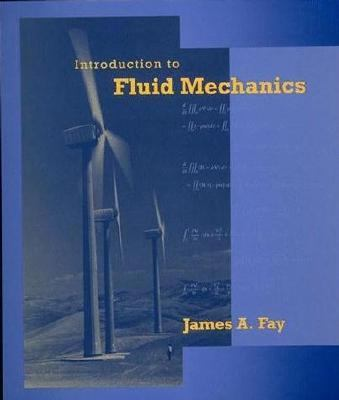147814

9780262061650

# Introduction to Fluid MechanicsOut of Stock

The item you're looking for is currently unavailable.

Most renters respond to questions in 48 hours or less.
The response will be emailed to you.
• ISBN-13: 9780262061650
• ISBN: 0262061651
• Publisher: MIT Press

Fay, James A.

### SUMMARY

Introduction to Fluid Mechanics is a mathematically efficient introductory text for a basal course in mechanical engineering. More rigorous than existing texts in the field, it is also distinguished by the choice and order of subject matter, its careful derivation and explanation of the laws of fluid mechanics, and its attention to everyday examples of fluid flow and common engineering applications. Beginning with the simple and proceeding to the complex, the text introduces the principles of fluid mechanics in orderly steps. At each stage practical engineering problems are solved, principally in engineering systems such as dams, pumps, turbines, pipe flows, propellers, and jets, but with occasional illustrations from physiological and meteorological flows. The approach builds on the student's experience with everyday fluid mechanics, showing how the scientific principles permit a quantitative understanding of what is happening and provide a basis for designing engineering systems that achieve the desired objectives. Introduction to Fluid Mechanics differs from most engineering texts in several respects: The derivations of the fluid principles (especially the conservation of energy) are complete and correct, but concisely given through use of the theorems of vector calculus. This saves considerable time and enables the student to visualize the significance of these principles. More attention than usual is given to unsteady flows and their importance in pipe flow and external flows. Finally, the examples and exercises illustrate real engineering situations, including physically realistic values of the problem variables. Many of these problems require calculation of numerical values, giving the student experience in judging the correctness of his or her numerical skills.Fay, James A. is the author of 'Introduction to Fluid Mechanics' with ISBN 9780262061650 and ISBN 0262061651.

You can find lots of answers to common customer questions in our FAQs

View a detailed breakdown of our shipping prices